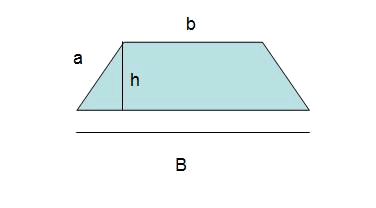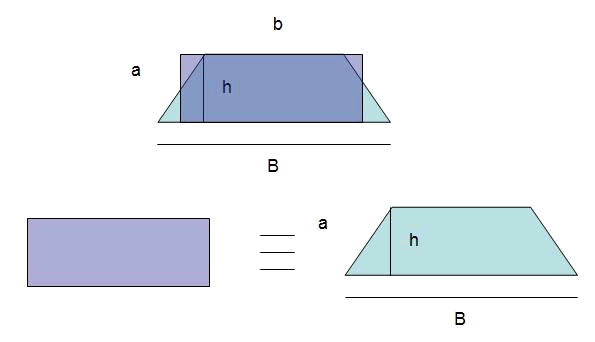# The trapezoidThe area of the trapezoid is: $$A=\frac{(B+b) \cdot h}{2}$$\$

With the following graph it is possible to interpret the previous formula:The term $$\dfrac{B+b}{2}$$ can be interpreted as the long side of the rectangle or the average of the upper and lower sides of the trapeze